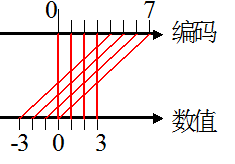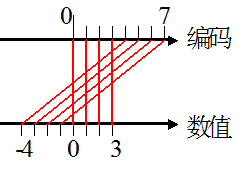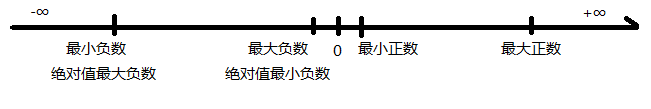## 期末总结系列1——数的表示

————————下面是一些开头的废话，可以不看————————

——————我是分割线，上面的废话可以不看，下面才是重点————————

1234     = 1 * 1000 + 2 * 100 + 3 * 10 + 4 * 1

= 1 * 10^3 + 2 * 10^2 + 3 * 10^1 + 4 * 10^0

0.4321 = 4 * 0.1 + 3 * 0.01 + 2 * 0.001 + 1 * 0.0001

= 4 * 10^-1 + 3 * 10^-2 + 2 * 10^-3 + 1 * 10^-4

1010.1001 = 1010 + 0.1001

1010      = 1 * 8 + 0 * 4 + 1 * 2 + 0 * 1

= 1 * 2^3 + 0 * 2^2 + 1 * 2^1 + 0 * 2^0

0.1001 = 0.1 + 0.00 + 0.000 + 0.0001

=1 * 2^-1 + 0 * 2^-2 + 0 * 2^-3 + 1 * 2^-4

 2^10 2^9 2^8 2^7 2^6 2^5 2^4 2^3 2^2 2^1 2^0 1024 512 256 128 64 32 16 8 4 2 1

 2^10 2^9 2^8 2^7 2^6 2^5 2^4 2^3 2^2 2^1 2^0 1024 512 256 128 64 32 16 8 4 2 1 1

 2^10 2^9 2^8 2^7 2^6 2^5 2^4 2^3 2^2 2^1 2^0 1024 512 256 128 64 32 16 8 4 2 1 1 1

 2^10 2^9 2^8 2^7 2^6 2^5 2^4 2^3 2^2 2^1 2^0 1024 512 256 128 64 32 16 8 4 2 1 1 1 1

 2^10 2^9 2^8 2^7 2^6 2^5 2^4 2^3 2^2 2^1 2^0 1024 512 256 128 64 32 16 8 4 2 1 1 1 1 1

 2^10 2^9 2^8 2^7 2^6 2^5 2^4 2^3 2^2 2^1 2^0 1024 512 256 128 64 32 16 8 4 2 1 1 1 1 1 1

 2^10 2^9 2^8 2^7 2^6 2^5 2^4 2^3 2^2 2^1 2^0 1024 512 256 128 64 32 16 8 4 2 1 1 0 0 1 1 0 1 0 0 1 0

0.4321 * 2 = 0.8642  整数部分为0

0.8642 * 2 = 1.7284  整数部分为1

0.7284 * 2 = 1.4568  整数部分为1

0.4568 * 2 = 0.9136  整数部分为0

0.9136 * 2 = 1.8272  整数部分为1

 原码 真值 0 0 0 0 0 1 0 0 1 1 2 0 1 0 2 3 0 1 1 3 4 1 0 0 -0 5 1 0 1 -1 6 1 1 0 -2 7 1 1 1 -3反码 真值 0 0 0 0 0 1 0 0 1 1 2 0 1 0 2 3 0 1 1 3 7 1 1 1 -0 6 1 1 0 -1 5 1 0 1 -2 4 1 0 0 -3补码 真值 0 0 0 0 0 1 0 0 1 1 2 0 1 0 2 3 0 1 1 3 0 0 0 0 0 7 1 1 1 -1 6 1 1 0 -2 5 1 0 1 -3

 补码 真值 0 0 0 0 0 1 0 0 1 1 2 0 1 0 2 3 0 1 1 3 0 0 0 0 0 7 1 1 1 -1 6 1 1 0 -2 5 1 0 1 -3 4 1 0 0 -4原码 真值 0 0 0 0 0.00 0.00 1 0 0 1 0.25 2^-2 2 0 1 0 0.50 2^-1 3 0 1 1 0.75 (2^-1)+(2^-2) 4 1 0 0 -0.00 -0.00 5 1 0 1 -0.25 -2^-2 6 1 1 0 -0.50 -2^-1 7 1 1 1 -0.75 -((2^-1)+(2^-2))

 反码 真值 0 0 0 0 0.00 0.00 1 0 0 1 0.25 2^-2 2 0 1 0 0.50 2^-1 3 0 1 1 0.75 (2^-1)+(2^-2) 7 1 1 1 -0.00 -0.00 6 1 1 0 -0.25 -2^-2 5 1 0 1 -0.50 -2^-1 4 1 0 0 -0.75 -((2^-1)+(2^-2))

 补码 真值 0 0 0 0 0.00 0.00 1 0 0 1 0.25 2^-2 2 0 1 0 0.50 2^-1 3 0 1 1 0.75 (2^-1)+(2^-2) 0 0 0 0 -0.00 -0.00 7 1 1 1 -0.25 -2^-2 6 1 1 0 -0.50 -2^-1 5 1 0 1 -0.75 -((2^-1)+(2^-2))

 补码 真值 0 0 0 0 0.00 0.00 1 0 0 1 0.25 2^-2 2 0 1 0 0.50 2^-1 3 0 1 1 0.75 (2^-1)+(2^-2) 0 0 0 0 -0.00 -0.00 7 1 1 1 -0.25 -2^-2 6 1 1 0 -0.50 -2^-1 5 1 0 1 -0.75 -((2^-1)+(2^-2)) 4 1 0 0 -1